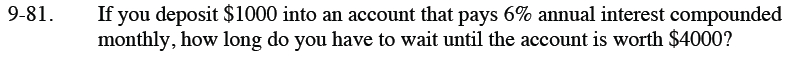Home > CCA2 > Chapter 9 > Lesson 9.3.1 > Problem9-81

9-81.

If you deposit $1000 into an account that pays 6% annual interest compounded monthly, how long do you have to wait until the account is worth$4000? Homework Help ✎See the Math Notes box in Appendix B, Lesson B.1.3.

$4000=1000\left(1+\frac{0.06}{12}\right)^{12t}$

$4=1.005^{12t}$

Take the log of both sides and then continue solving.